# Pressure Pressure A force can also change the

• Slides: 20PressurePressure �A force can also change the shape of something, as well as changing it’s motion.Pressure �A force can also change the shape of something, as well as changing it’s motion. � Eg – if someone stands on soft, wet sand, their feet may sink into it. The weight force of the person has changed the shape of the surface.Pressure �A force can also change the shape of something, as well as changing it’s motion. � Eg – if someone stands on soft, wet sand, their feet may sink into it. The weight force of the person has changed the shape of the surface. � Pressure depends on the area over which a force is acting.Pressure � Pressure = force area or P=F APressure � Units - = force area or P=F A for pressure include: Newtons per square metre N m-2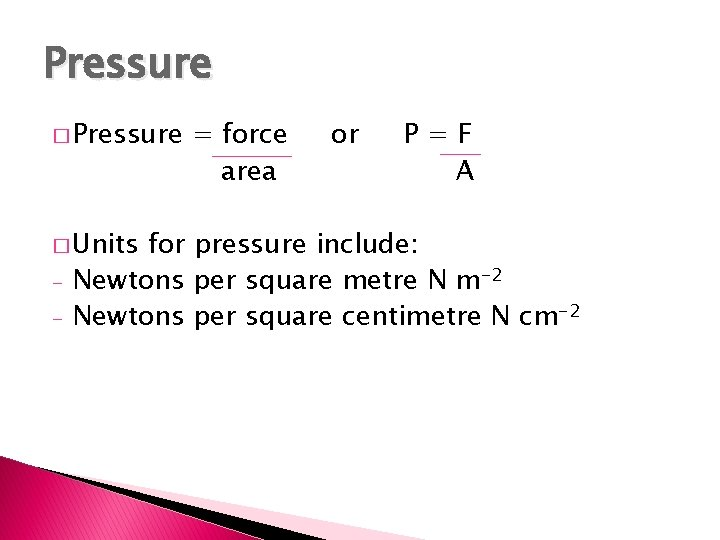Pressure � Units - = force area or P=F A for pressure include: Newtons per square metre N m-2 Newtons per square centimetre N cm-2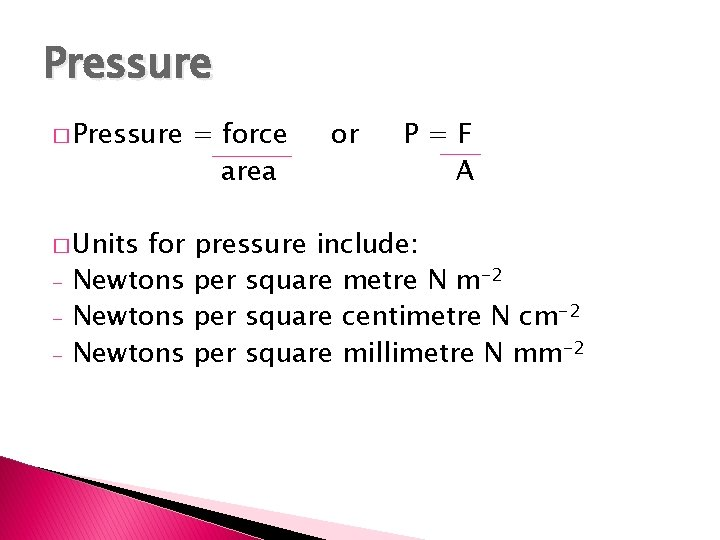Pressure � Pressure = force area � Units pressure include: per square metre N m-2 per square centimetre N cm-2 per square millimetre N mm-2 - for Newtons or P=F A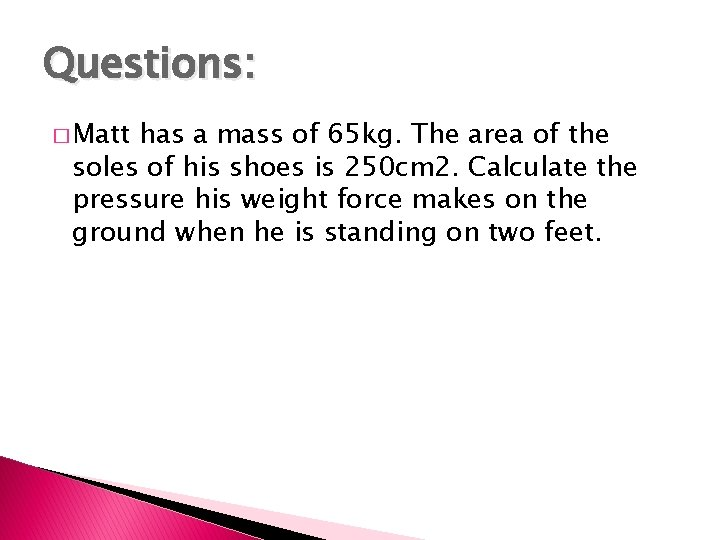Questions: � Matt has a mass of 65 kg. The area of the soles of his shoes is 250 cm 2. Calculate the pressure his weight force makes on the ground when he is standing on two feet.Questions: � Matt has a mass of 65 kg. The area of the soles of his shoes is 250 cm 2. Calculate the pressure his weight force makes on the ground when he is standing on two feet. � To find Matt’s weight, use weight = 10 x mass = 10 x 65 = 650 N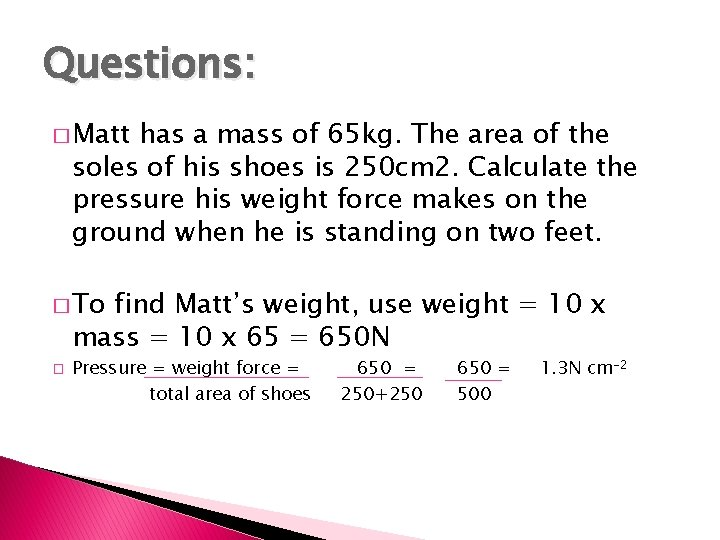Questions: � Matt has a mass of 65 kg. The area of the soles of his shoes is 250 cm 2. Calculate the pressure his weight force makes on the ground when he is standing on two feet. � To find Matt’s weight, use weight = 10 x mass = 10 x 65 = 650 N � Pressure = weight force = total area of shoes 650 = 250+250 650 = 500 1. 3 N cm-2Pressure F P A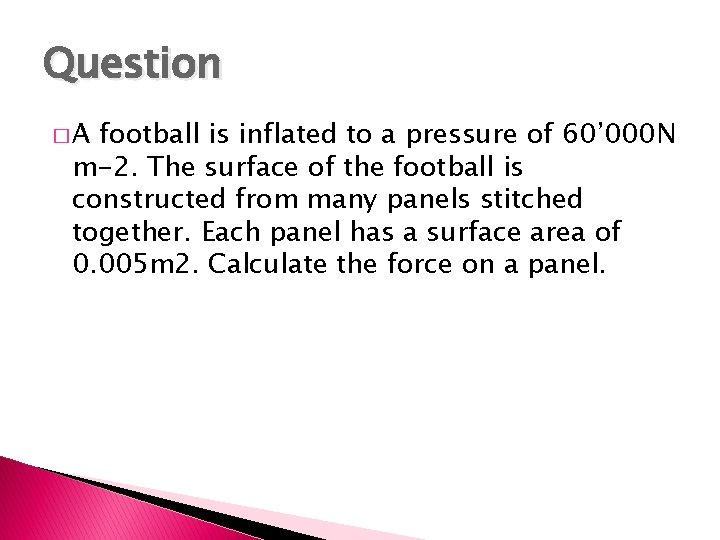Question �A football is inflated to a pressure of 60’ 000 N m-2. The surface of the football is constructed from many panels stitched together. Each panel has a surface area of 0. 005 m 2. Calculate the force on a panel.Question �A football is inflated to a pressure of 60’ 000 N m-2. The surface of the football is constructed from many panels stitched together. Each panel has a surface area of 0. 005 m 2. Calculate the force on a panel. � F=PA. 60’ 000 x 0. 005 = 300 N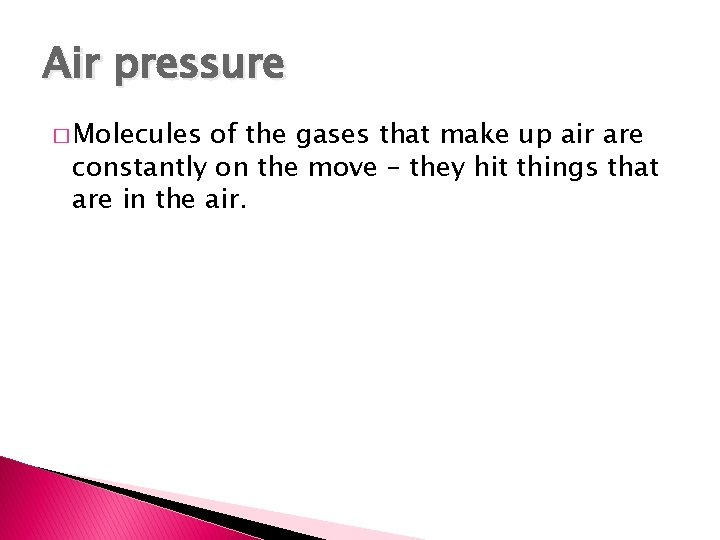Air pressure � Molecules of the gases that make up air are constantly on the move – they hit things that are in the air.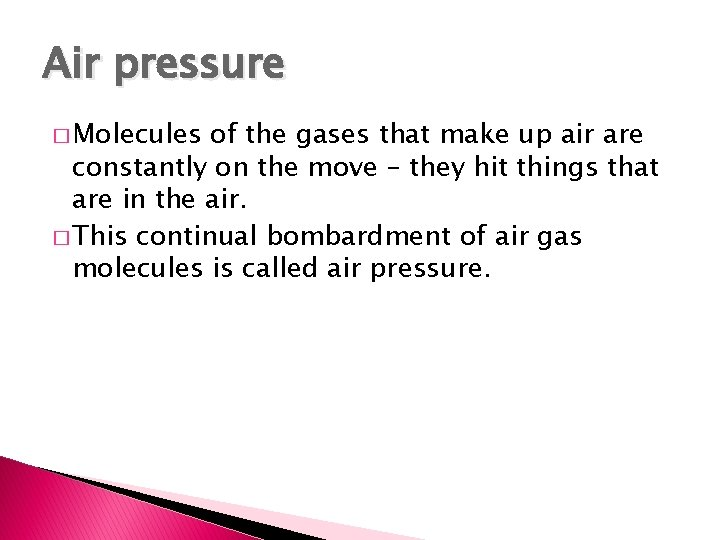Air pressure � Molecules of the gases that make up air are constantly on the move – they hit things that are in the air. � This continual bombardment of air gas molecules is called air pressure.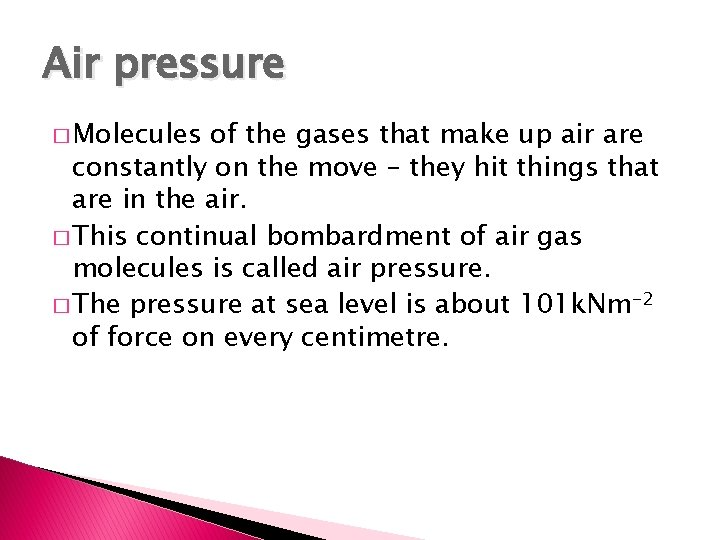Air pressure � Molecules of the gases that make up air are constantly on the move – they hit things that are in the air. � This continual bombardment of air gas molecules is called air pressure. � The pressure at sea level is about 101 k. Nm-2 of force on every centimetre.Air pressure � Molecules of the gases that make up air are constantly on the move – they hit things that are in the air. � This continual bombardment of air gas molecules is called air pressure. � The pressure at sea level is about 101 k. Nm-2 of force on every centimetre. � Living creatures on earth have adapted to this pressure and it isn’t noticed.Questions 1. A 65 kg skier stands with her weight evenly distributed between her two skis, each of which has a mass of 2. 1 kg. Each ski has a surface area in contact with the snow of 0. 15 m 2. Calculate the pressure on the snow.Question 1. a) b) c) d) Arapeta has a mass of 70 kg and is wearing rugby boots. Each shoe has 7 studs on its sole. Each stud has a surface area of 2 cm 2 Change 2 cm 2 to metres squared Calculate the pressure on a hard surface from the studs if he is standing on both feet. Explain how the pressure on the surface changes if he is standing on one foot only Explain what advantage he will have when he is running on a slippery surface wearing these boots compared to shoes without studs.